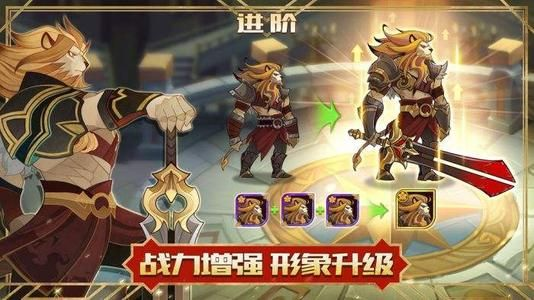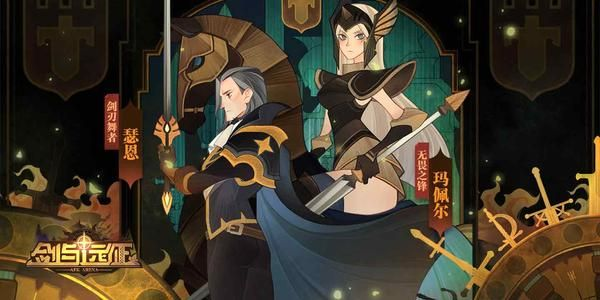### 剑与远征神话英雄进阶材料需要多少？各等级英雄合成消耗表[多图]

2020-4-26 13:36:42小编：zyh我要评论(如：一个稀有品质的英雄进阶到稀有+，需要消耗掉2个稀有品质的自己。)《剑与远征》各级英雄合成费用分析

30元=300碎片=5个稀有=5个稀有×6元

3个稀有=1个稀有➕=18元

3个稀有➕=1个精英=3×18=54元

2个精英=1个精英➕=2×54=108元

3个精英➕=1个史诗=3×108=324元

1个史诗+1个精英➕=1个史诗➕=324+108=432元人民币。

1个史诗➕+1个史诗➕=1个传说=324+324=648元

1个传说+1个史诗➕=1个传说➕=648+432=1080元

1个传说➕+2个精英➕=1个神话=1080+2×108=1296元• 相关游戏
• 相关文章
• 发表评论